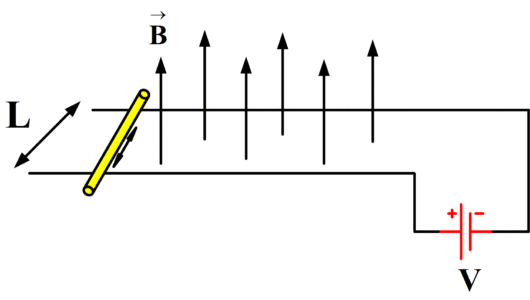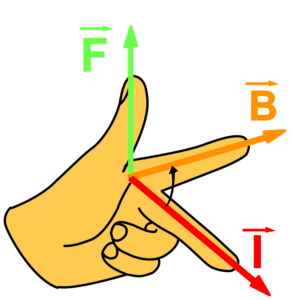# Magnetic Force on a Current Carrying Conductor

Want create site? Find Free WordPress Themes and plugins.

A current carrying conductor produces its own magnetic field. Such a conductor is placed in a magnetic field. The magnetic field of the conductor will interact with the external magnetic field. As a result of which the conductor may experience a force. It can be observed by the following experiment.

Consider a copper rod which can move on a pair of copper rails. The whole arrangement is placed in a uniform magnetic field. The magnetic field on the rod is directed vertically upwards as shown in fig.When a current is passed through a copper rod from a batter, a rod moves on the rails. The relative direction of current, magnetic field and motion of conductor (force) are shown in the figure.It can be observed that the force on a conductor is always at right angle to the plane containing the rod and the direction of the magnetic field B and is predicted by Fleming’s Left-Hand rule. The magnitude of the force acting on the rod depends on the following factors.

• The force F is directly proportional to Sin? where ? is the angle between conductor L and magnetic field B.

$F\propto Sin\alpha$

It means that the force is zero if the rod is placed parallel to the magnetic field and force is maximum when the conductor is placed at right angle to a field.

• The force F is directly proportional to the current I flowing through the conductor.

$F\propto ~I$

• The force F is directly proportional to the length of the conductor L inside the magnetic field B.

$F\propto ~L$

• The force F is directly proportional to the strength of magnetic field applied.

$F\propto B$

Combining all four factors, we can write a simple following equation

$F\propto BIL~Sin\alpha$

Or

$F=KBIL~Sin\alpha$

Where K is the constant of proportionality. The SI units of force, current, conductor length and magnetic field are given below:

Force is measured in Newton (N)

Current is measured in ampere (A)

Conductor length is in Meter (m)

Magnetic field strength is measured in Tesla Whereas;

$Tesla={}^{N}/{}_{Am}$

By putting all units is above formula, we can observe that K has no units.

Example

A 20 cm wire carrying a current of 10 A is placed in a uniform magnetic field of 0.3 Tesla. If the wire makes an angle of 40o with the direction of magnetic field, find the magnitude of the force acting on the wire?

Solution

Length of the wire = L= 20 cm = 0.2 m

Current in the wire = I= 10 A

Strength of magnetic field = B = 0.30 T

Angle along magnetic field =α= 40o

In order to calculate Force, we have following formula:

$F=BIL~Sin\alpha$

So,

$F=0.30*10*0.2*Sin~({{40}^{o}})$

$F=0.386~N$

Did you find apk for android? You can find new Free Android Games and apps.